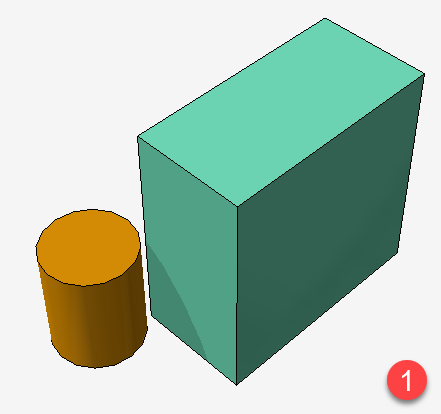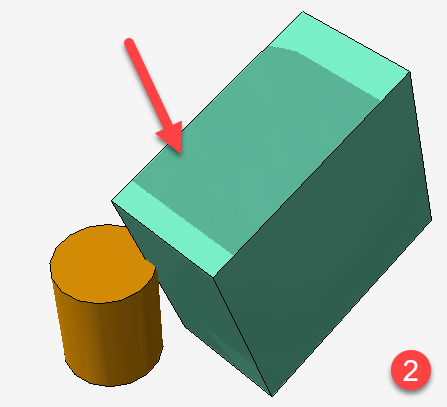# Broken shadow maps after a modelView multiplication

Hello,

We have a perfectly working shadow map setup.

Under certain conditions, one object in the scene needs to be drawn roto-translated by a 4x4 matrix.

We resolved calling:

``````glPushMatrix();
glMultMatrix(xForm); // modelView matrix multiplication
Draw();
glPopMatrix();
``````

The result is a completely messed up shadows on the roto-translated object itself.

I cannot understand where the problem is and if we can fix it. Is it possible that during the first pass drawing, with the camera on the directional light position, the object is roto-translated to a wrong position compared to what happens in the second pass with the actual scene camera?

What else should I check?

Thanks,

Alberto

• Are you applying the same transformation for both passes?
• Are you applying the transformation to the model-view matrix (in both cases)?
• In the second pass, you have to transform the vertices to both the light space (to determine the coordinates for the shadow map lookup) and the camera space; is the transformation being applied to both?
• Is the transformation being taken into account when computing the bounds of the shadow map? Or is it pushing the object outside of those bounds?

• Are you applying the same transformation for both passes?

Yes, as you can see in the pseudocode below.

• Are you applying the transformation to the model-view matrix (in both cases)?

Yes, as you can see in the pseudocode below.

• In the second pass, you have to transform the vertices to both the light space (to determine the coordinates for the shadow map lookup) and the camera space; is the transformation being applied to both?

I’m not sure about this. I’m only doing what you see in the pseudocode below. Maybe this is my problem?

• Is the transformation being taken into account when computing the bounds of the shadow map? Or is it pushing the object outside of those bounds?

I’m not sure about this. I’m only doing what you see in the pseudocode below. Maybe this is my problem?

Pseudocode

Custom Mesh class:

``````    class MyMesh : Mesh
{
public Transformation t = new Identity();

public MyMesh(Point3D[] vertices, Triangle[] triangles) : base(vertices, triangles)
{
}

protected override void Draw(Params data)
{
data.RenderContext.PushModelView();
data.RenderContext.MultMatrixModelView(t);
base.Render(data);
data.RenderContext.PopModelView();
}

{
data.RenderContext.PushModelView();
data.RenderContext.MultMatrixModelView(t);
data.RenderContext.PopModelView();
}

protected override void DrawEdges(Params data)
{
data.RenderContext.PushModelView();
data.RenderContext.MultMatrixModelView(t);
base.DrawEdges(data);
data.RenderContext.PopModelView();
}
}
``````

Scene objects setup:

`````` MyMesh m = MyMesh.CreateBox(100, 200, 200);

m.t = new Translation(0, 20, -10) * new Rotation(PI/10, xAxis);

Mesh m1 = Mesh.CreateCylinder(40, 100, 16);

m1.Translate(-20,-40,0);

``````

Image 1) ‘t’ is identityImage 2) ‘t’ is a roto-translation transformation. The arrow indicates the wrong shadow map.Nothing in the pseudo-code stands out. From the bottom image, it appears that the shadow map is rendered using the transformed cube but the lookup is being performed with the un-transformed coordinates. IOW:

It appears that the transformation is only applied to the camera-space coordinates, and not the light-space coordinates.

Also: you should try to get a single shadow map working before progressing to cascaded (parallel-split) shadow maps.

That article assumes you know how shadow mapping works. Note the mentions of “light’s view-projection transformation matrix” (or transform), textureMatrix, texScaleBiasMatrix, etc.

For a decent overview on how this works, see the diagram and description in the Projective Texturing section here:

One you understand it though, just pass the matrices into your render-pass GLSL shaders as you would any other matrices. Ignore the mentions of the old GL fixed-function texture coord generation method of doing the same – e.g. `glTexGen*()`, `EYE_LINEAR`, etc.

Thanks GClements,

The problem was exactly the one you supposed. I was facilitated from seeing everything working properly turning shaders off.

Now I update the transformation (‘t’) used by the shader with the following call and the result is perfect!

``````glUniformMatrix4fvARB(-1, 1, false, t);
``````

Thanks again,

Alberto

Why are you using -1 for the location ?

From this page, it is said that for -1, the uniform will not be changed.

Ah sorry. I’ve copied in the post the default value but actually in the code you get it this way:

``````locTransformMatrix = GetUniformLocation("matrix")

glUniformMatrix4fvARB(locTransformMatrix, 1, false, t);
``````

Thanks again,

Alberto